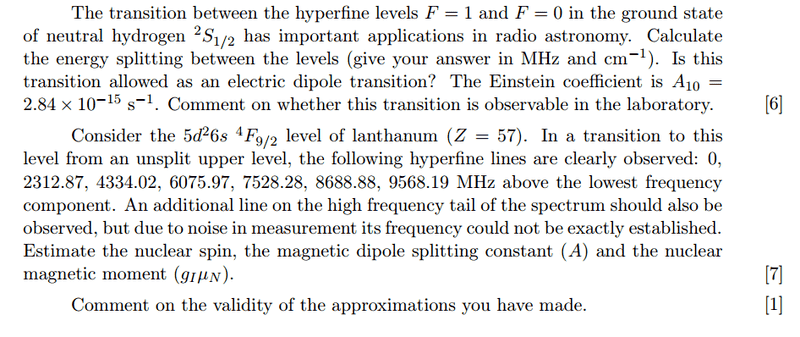# Hyperfine Interaction Splitting

• unscientific

## Homework Statement

(a)Find splitting between F=0 and F=1 in hydrogen
(b) Find the constant ##A## and nuclear magnetic moment## The Attempt at a Solution

Hyperfine splitting is given by:

$$H_{hf} = \frac{A}{2} \left[ F(F+1) - I(I+1) - J(J+1) \right]$$

The intervals are given by:
$$\Delta E_{hf} = E_F - E_{F-1} = AF$$

Part(a)

Using ##G_I = 5.6##, ##I= \frac{1}{2}## for a single proton, ##n = 1, Z = 1## I get splittings as ## 1.42~ MHz## and ##474.6 ~cm^{-1}##. Could somebody verify this?

Part (b)

For ##J = \frac{9}{2}##, it implies that ##I = \frac{1}{2}## for the energy levels to be split into 6 levels, ##F= I + J = 0,1,2,3,4,5##.

Using the interval rule, the energy intervals are ##A, 2A, 3A, 4A, 5A##.

Taking the maximum energy - minimum energy observed = energy spacing between F=0 and F=5.

$$15 A = 10^9 h (9568.19-2312.87)$$

This gives ##A = 3.21 \times 10^{-22}## and ##g_i \mu_N = 3.65 \times 10^{-23}##.

However, this means that ##g_I = 7200##. Is this reasonable?

Your answers in (a) are inconsistent by a factor of 10, and both are wrong by some power of 10.
unscientific said:
Taking the maximum energy - minimum energy observed = energy spacing between F=0 and F=5.
I don't think that makes sense. Those photon energies are differences between states already, not the absolute energy of something.
Also, where does the factor of 109 come from?

mfb said:
Your answers in (a) are inconsistent by a factor of 10, and both are wrong by some power of 10.
I don't think that makes sense. Those photon energies are differences between states already, not the absolute energy of something.
Also, where does the factor of 109 come from?

I'm thinking consider a transition from the upper level to the F=5 level vs the transition from the upper level to the F=0 level. The difference in energy observed would be the spacing between F=0 and F=5 levels, which is 15A.